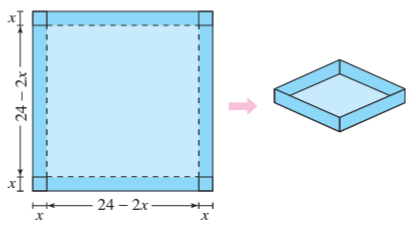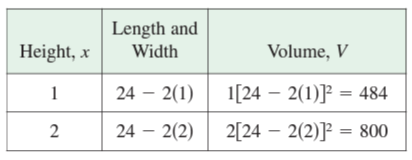×
Get Full Access to Calculus: Early Transcendental Functions - 6 Edition - Chapter 4.7 - Problem 2
Get Full Access to Calculus: Early Transcendental Functions - 6 Edition - Chapter 4.7 - Problem 2

×

# ?Numerical, Graphical, and Analytic Analysis An open box of maximum volume is to be made from a square piece of material,24 inches on a side,by cuttingISBN: 9781285774770 141

## Solution for problem 2 Chapter 4.7

Calculus: Early Transcendental Functions | 6th Edition

• Textbook Solutions
• 2901 Step-by-step solutions solved by professors and subject experts
• Get 24/7 help from StudySoup virtual teaching assistantsCalculus: Early Transcendental Functions | 6th Edition

4 5 1 424 Reviews
10
3
Problem 2

Numerical, Graphical, and Analytic Analysis An open box of maximum volume is to be made from a square piece of material,24 inches on a side,by cutting equal squares from the corners and turning up the sides (see figure).(a) Analytically complete six rows of a table such as the one below. (The first two rows are shown.) Use the table to guess the maximum volume.(b) Write the volume V as a function of x.

(c) Use calculus to find the critical number of the function in part (b) and find the maximum value.

(d) Use a graphing utility to graph the function in part (b) and verify the maximum volume from the graph.

Step-by-Step Solution:

Step 1 of 5) Exploration In Example 7, the rate of conversion was assumed to be proportional to the square of the unconverted amount. How would the result change if the rate of conversion were assumed to be proportional to the unconverted amount

Step 2 of 2

##### ISBN: 9781285774770

Since the solution to 2 from 4.7 chapter was answered, more than 238 students have viewed the full step-by-step answer. Calculus: Early Transcendental Functions was written by and is associated to the ISBN: 9781285774770. The full step-by-step solution to problem: 2 from chapter: 4.7 was answered by , our top Calculus solution expert on 11/14/17, 10:53PM. This textbook survival guide was created for the textbook: Calculus: Early Transcendental Functions, edition: 6. This full solution covers the following key subjects: . This expansive textbook survival guide covers 134 chapters, and 10738 solutions. The answer to “?Numerical, Graphical, and Analytic Analysis An open box of maximum volume is to be made from a square piece of material,24 inches on a side,by cutting equal squares from the corners and turning up the sides (see figure). (a) Analytically complete six rows of a table such as the one below. (The first two rows are shown.) Use the table to guess the maximum volume. (b) Write the volume V as a function of x.(c) Use calculus to find the critical number of the function in part (b) and find the maximum value.(d) Use a graphing utility to graph the function in part (b) and verify the maximum volume from the graph.” is broken down into a number of easy to follow steps, and 112 words.

## Discover and learn what students are asking

Unlock Textbook Solution